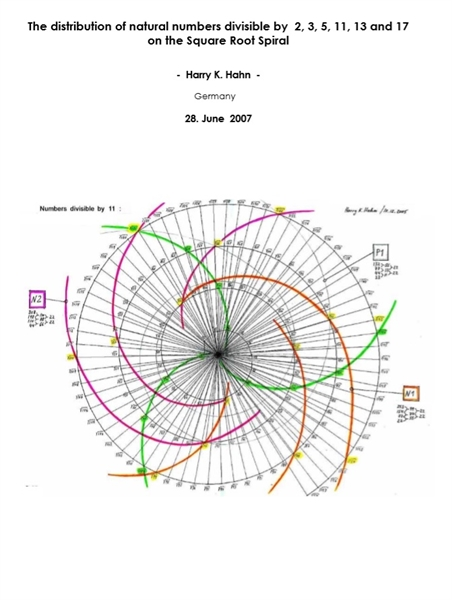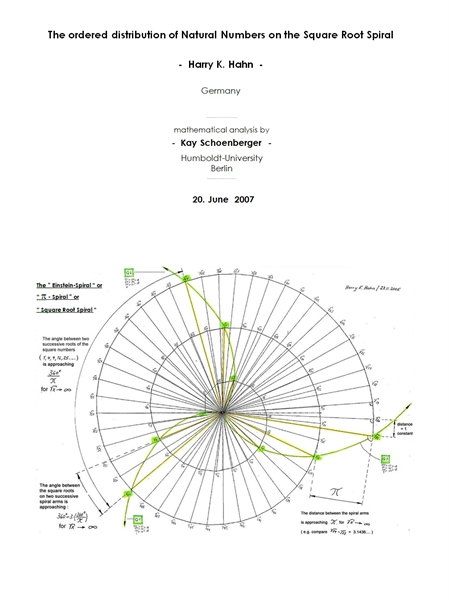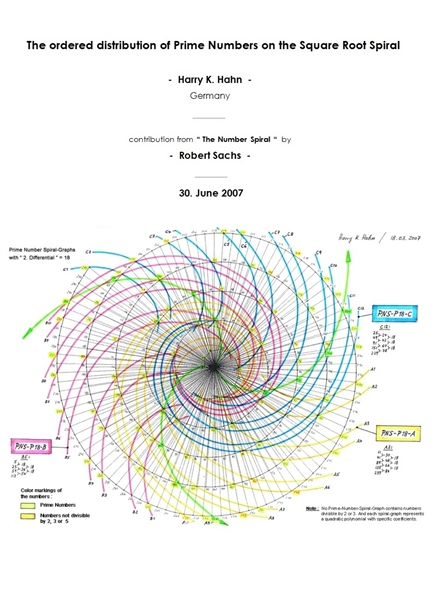My Account |   | Help
• Harry HahnGeneralInfo
General Information:•The Distribution of Natural Numbers Divisible by 2, 3, 5, 11, 13 a...

By: Harry K. Hahn; Harry K. Hahn

The Square Root Spiral ( or “Spiral of Theodorus” or “Einstein-Spiral” ) is a very interesting geometrical structure, in which the square roots of all natural numbers have a defined (spatial ) position to each other. The Square Root Spiral develops from a right angled base triangle with the two legs ( cathets ) having the length 1, and with the long side ( hypotenuse ) having a length which is equal to the square root of 2. The Square Root Spiral is formed by further...

From the image FIG 1 it is evident that the numbers divisible by 2 ( marked in yellow ) lie on defined spiral graphs which have their starting point in or near the centre of the square root spiral. These spiral graphs have either a positive or a negative rotation direction. A spiral graph which has a clockwise rotation direction shall be called negative (N) and a spiral graph which has an counterclockwise rotation direction shall be called positive (P). The gre...

•The Ordered Distribution of Natural Numbers on the Square Root Spiral

By: Harry K. Hahn

The Square Root Spiral ( or “Wheel of Theodorus” or “Einstein Spiral” or “Root Snail” ) is a very interesting geometrical structure, in which the square roots of all natural numbers have a clear defined spatial orientation to each other. This enables the attentive viewer to find many interdependencies between natural numbers, by applying graphical analyses techniques. Therefore the square root spiral should be an important research object for all professionals working ...

Another striking property of the Square Root Spiral is the fact, that the square roots of all square numbers ( 4, 9, 16, 25, 36… ) lie on 3 highly symmetrical spiral graphs which divide the square root spiral into 3 equal areas. ( see FIG.1 : graphs Q1, Q2 and Q3 drawn in green color ).

•The Distribution of Prime Numbers on the Square Root Spiral

By: Harry K. Hahn

The Square Root Spiral ( or “Spiral of Theodorus” or “Einstein Spiral” ) is a very interesting geometrical structure in which the square roots of all natural numbers have a clear defined orientation to each other. This enables the attentive viewer to find many spatial interdependencies between natural numbers, by applying simple graphical analysis techniques. Therefore the Square Root Spiral should be an important research object for professionals, who work in the fiel...

Another striking property of the Square Root Spiral is the fact, that the square roots of all square numbers ( 4, 9, 16, 25, 36… ) lie on 3 highly symmetrical spiral graphs which divide the square root spiral into 3 equal areas. ( see FIG.1 : graphs Q1, Q2 and Q3 drawn in green color ).